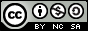- 目录 -
LeetCode 440. K-th Smallest in Lexicographical Order(思路)

Given integers n and k, find the lexicographically k-th smallest integer in the range from 1 to n.

Note: 1 ≤ k ≤ n ≤ 109.

Example:

Input:
n: 13   k: 2

Output:
10

Explanation:
The lexicographical order is [1, 10, 11, 12, 13, 2, 3, 4, 5, 6, 7, 8, 9], so the second smallest number is 10.


## 代码

  1 2 3 4 5 6 7 8 9 10 11 12 13 14 15 16 17 18 19 20 21 22 23 24 25 26 27 28 29 30 31  class Solution { public: int findKthNumber(int n, int k) { if (k > n) return -1; int cur = 1; k--; while (k) { long long sum = 0, l = cur, r = cur + 1; while (l <= n) { sum += min((long long)n + 1, r) - l; l *= 10, r *= 10; } if (sum <= k) { k -= sum; cur++; } else { cur *= 10; k--; } } return cur; } };